Locating and Ordering Fractions and Mixed Numbers on the Number Line

Learning Outcomes

• Locate and label improper and proper fractions on a number line
• Order fractions and mixed numbers on a number line
• Use inequality symbols to compare fractions and mixed numbers

Now we are ready to plot fractions on a number line. This will help us visualize fractions and understand their values.

Let us locate ${\Large\frac{1}{5},\frac{4}{5}},3,3{\Large\frac{1}{3},\frac{7}{4},\frac{9}{2}},5$, and ${\Large\frac{8}{3}}$ on the number line.

We will start with the whole numbers $3$ and $5$ because they are the easiest to plot.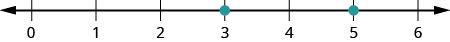The proper fractions listed are ${\Large\frac{1}{5}}$ and ${\Large\frac{4}{5}}$. We know proper fractions have values less than one, so ${\Large\frac{1}{5}}$ and ${\Large\frac{4}{5}}$ are located between the whole numbers $0$ and $1$. The denominators are both $5$, so we need to divide the segment of the number line between $0$ and $1$ into five equal parts. We can do this by drawing four equally spaced marks on the number line, which we can then label as ${\Large\frac{1}{5},\frac{2}{5},\frac{3}{5}}$, and ${\Large\frac{4}{5}}$.

Now plot points at ${\Large\frac{1}{5}}$ and ${\Large\frac{4}{5}}$.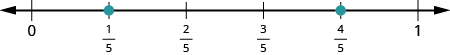The only mixed number to plot is $3{\Large\frac{1}{3}}$. Between what two whole numbers is $3{\Large\frac{1}{3}}$? Remember that a mixed number is a whole number plus a proper fraction, so $3{\Large\frac{1}{3}}>3$. Since it is greater than $3$, but not a whole unit greater, $3{\Large\frac{1}{3}}$ is between $3$ and $4$. We need to divide the portion of the number line between $3$ and $4$ into three equal pieces (thirds) and plot $3{\Large\frac{1}{3}}$ at the first mark.Finally, look at the improper fractions ${\Large\frac{7}{4},\frac{9}{2}}$, and ${\Large\frac{8}{3}}$. Locating these points will be easier if you change each of them to a mixed number.

${\Large\frac{7}{4}}=1{\Large\frac{3}{4}},{\Large\frac{9}{2}}=4{\Large\frac{1}{2}},{\Large\frac{8}{3}}=2{\Large\frac{2}{3}}$

Here is the number line with all the points plotted.Example

Locate and label the following on a number line: ${\Large\frac{3}{4},\frac{4}{3},\frac{5}{3}},4{\Large\frac{1}{5}}$, and ${\Large\frac{7}{2}}$.

Solution:
Start by locating the proper fraction ${\Large\frac{3}{4}}$. It is between $0$ and $1$. To do this, divide the distance between $0$ and $1$ into four equal parts. Then plot ${\Large\frac{3}{4}}$.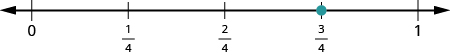Next, locate the mixed number $4{\Large\frac{1}{5}}$. It is between $4$ and $5$ on the number line. Divide the number line between $4$ and $5$ into five equal parts, and then plot $4{\Large\frac{1}{5}}$ one-fifth of the way between $4$ and $5$ .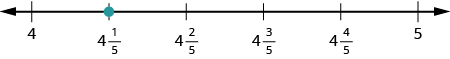Now locate the improper fractions ${\Large\frac{4}{3}}$ and ${\Large\frac{5}{3}}$. It is easier to plot them if we convert them to mixed numbers first.

${\Large\frac{4}{3}}=1{\Large\frac{1}{3}},{\Large\frac{5}{3}}=1{\Large\frac{2}{3}}$
Divide the distance between $1$ and $2$ into thirds.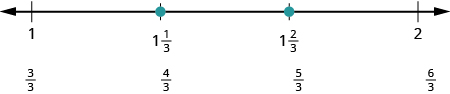Next let us plot ${\Large\frac{7}{2}}$. We write it as a mixed number, ${\Large\frac{7}{2}}=3{\Large\frac{1}{2}}$ . Plot it between $3$ and $4$.The number line shows all the numbers located on the number line.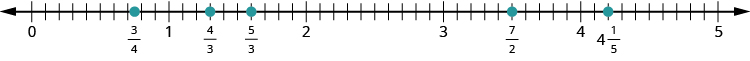try it

Watch the following video to see more examples of how to locate fractions on a number line.